# Intuition Behind Completing the Square

Hidden inside of every quadratic, there is a perfect square.

See below for the video version of this post:

In this post, I’m going to explain a very simple way to think about completing the square. The main idea behind completing the square is that hidden inside of every quadratic, there is a perfect square. To illustrate: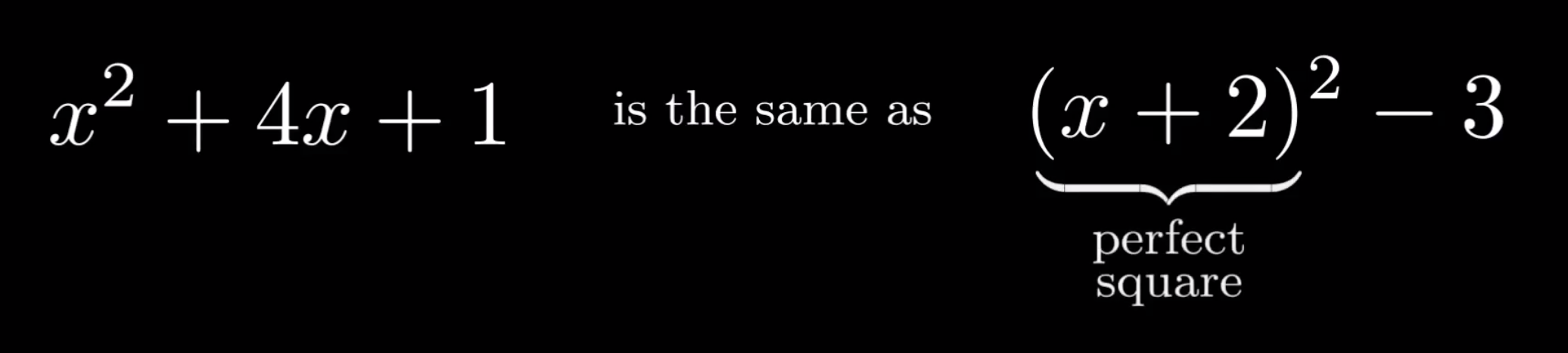Although these expressions may look different, they are actually the same. We can see this by expanding out the perfect square – indeed, we reach the original expression.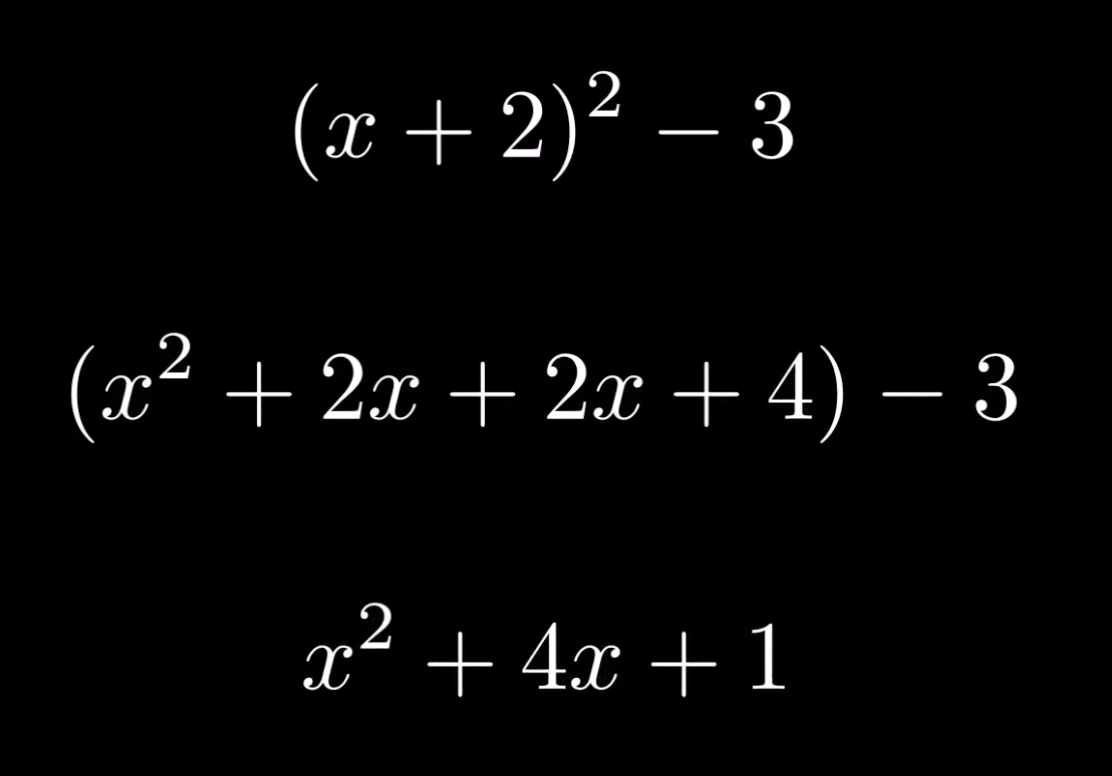However, this perfect square was simply given to us. We didn’t have to actually find the perfect square – we just had to verify that it was indeed the same as the original expression. In general, how do we find the perfect square that hides within any given quadratic expression?

The answer is to notice a relationship between the number inside a perfect square and the number on the $x$ in a quadratic expression. In general, the number in the square is half the number on the $x$.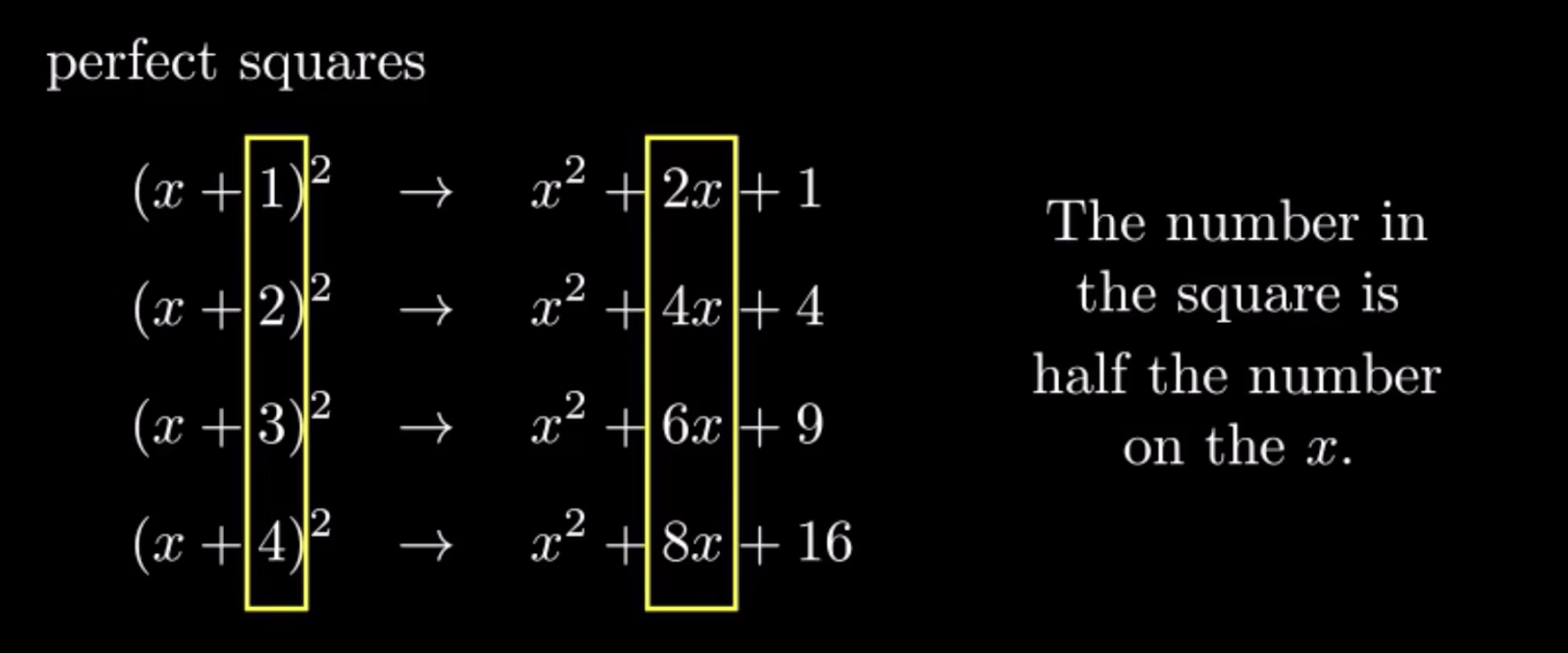Now, let’s work an example. Let’s complete the square on the expression $x^2+6x+13$, using the fact that the number in the square is half the number on the $x$. The number on the $x$ ix $6$, and half of that is $3$, so the perfect square must be $(x+3)^2$. When we expand out that perfect square, we get a result of $x^2+3x+3x+9$, which simplifies to $x^2+6x+9$, which is pretty close to the original expression $x^2+6x+13$.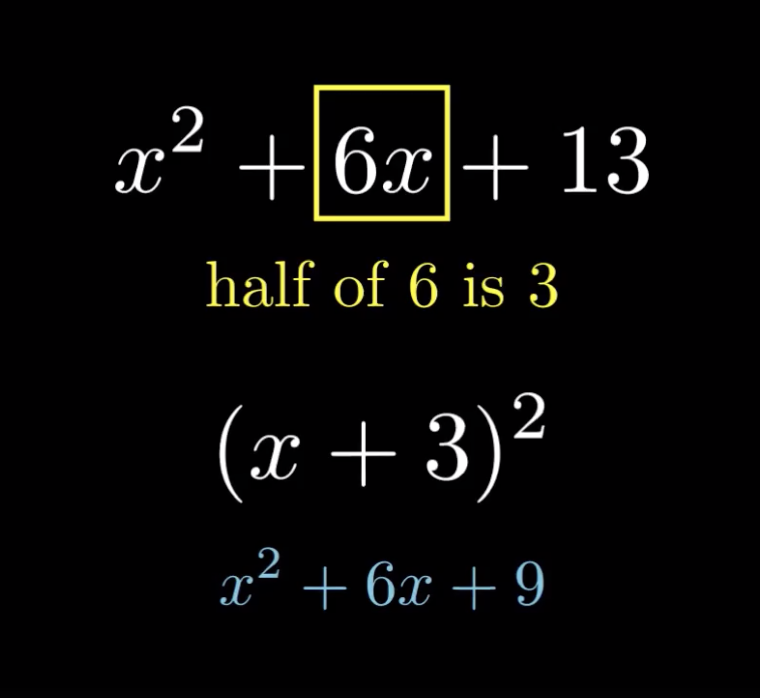The only adjustment we need to make is that we need to turn the $+9$ into a $+13$ so that it matches the original expression. So, we just need to add $4$ to the perfect square.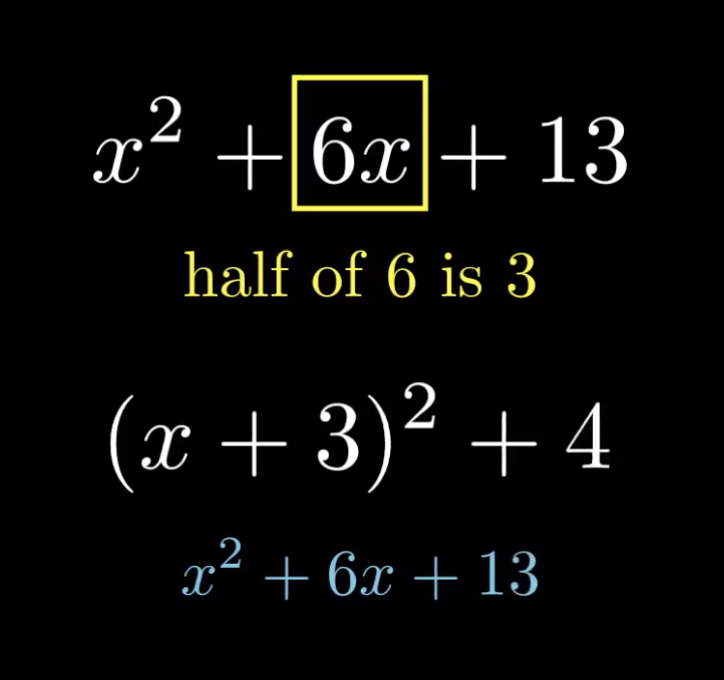And there we have it! We’ve completed the square.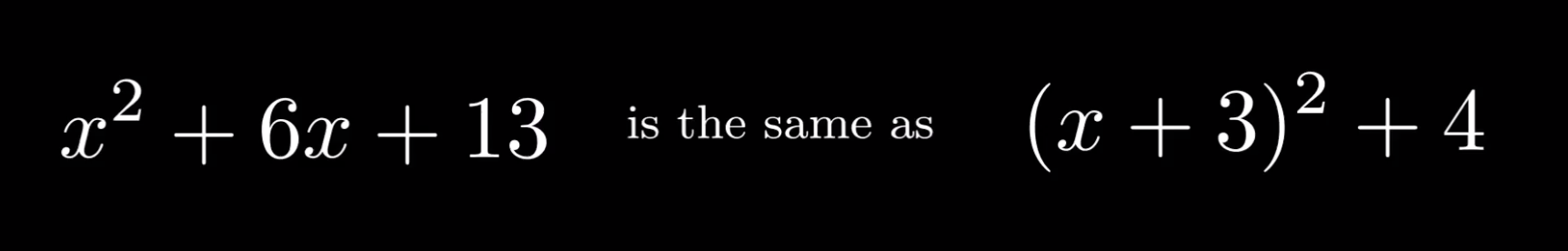Let’s work one more example: $x^2-10x+18$. This time, the number on the $x$ is $10$, and half of that is $5$ – and since the $x$ term is negative, we need to use subtraction within the perfect square: $(x-5)^2$. When we expand out that perfect square, we get a result of $x^2-5x-5x+25$, which simplifies to $x^2-10x+25$, which is pretty close to the original expression $x^2-10x+18$.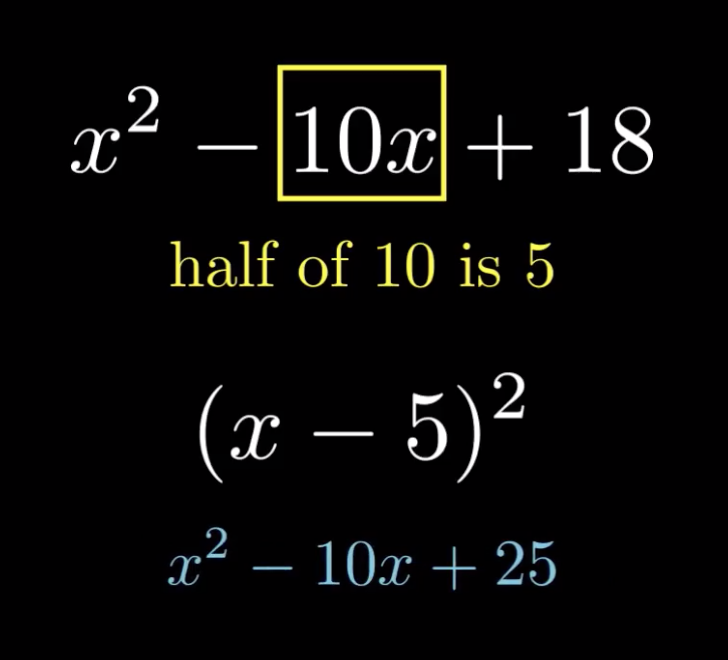The only adjustment we need to make is that we need to turn the $+25$ into a $+18$ so that it matches the original expression. So, we just need to subtract $7$ from the perfect square.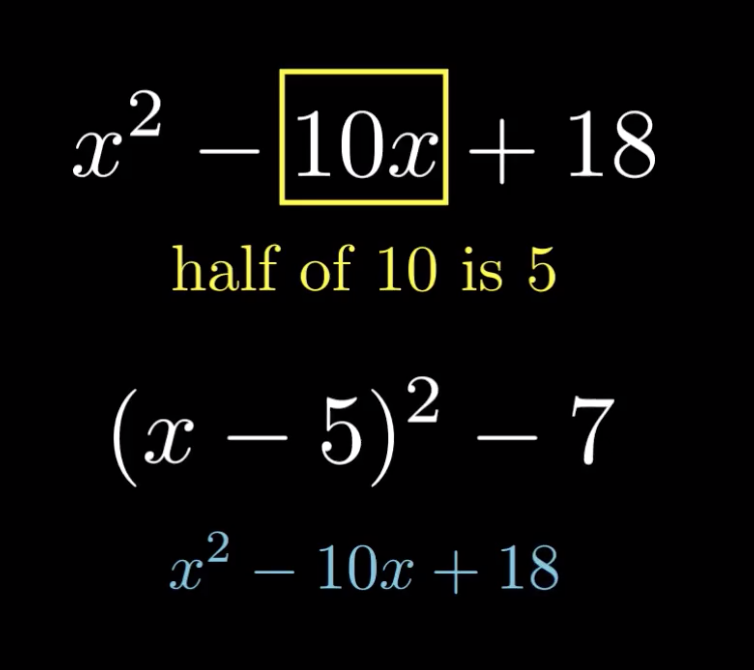And there we have it! We’ve completed the square.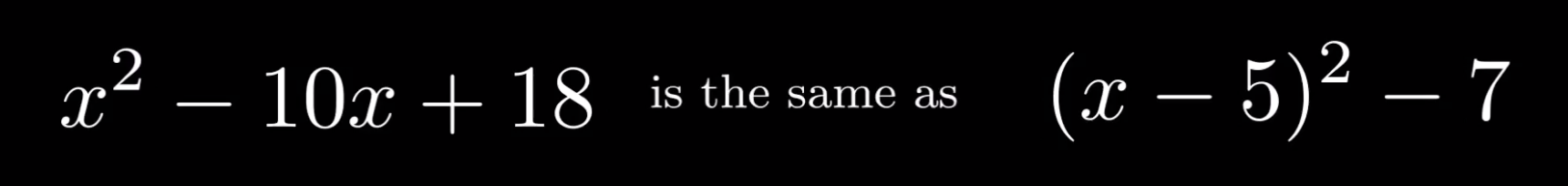Tags: